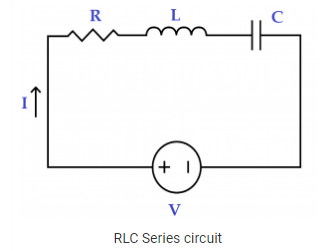Checkout NEET 2022 Question Paper Analysis : Checkout NEET 2022 Question Paper Analysis :

# RLC Circuit MCQs for NEET

The circuit consisting of a resistor (R), an inductor (L) and a capacitor (C) connected in series or parallel is called RLC circuit.Q1: A 6.8 kΩ resistor, a 7 mH coil, and a 0.02 μF capacitor are in parallel across a 17 kHz ac source. The coil’s internal resistance Rw is 30 Ω. The equivalent parallel resistance, Rp (eq) is

1. 1,878 Ω
2. 18,780 Ω
3. 18,750 Ω
4. 626 Ω

Q2: A 15 kΩ resistor, a 220 μH coil, and a 60 pF capacitor are in series across an ac source. What is the bandwidth of the circuit?

1. 138 MHz
2. 10,866 Hz
3. 1,907 Hz
4. 138 kHz

Q3: A 90 Ω resistor, a coil with 30 Ω of reactance, and a capacitor with 50 Ω of reactance are in series across a 12 V ac source. The current through the resistor is

1. 9 mA
2. 90 mA
3. 13 mA
4. 130 mA

Q4: The total impedance, expressed in polar form for a certain series RLC circuit with a 200 Hz, 15 V ac source has the following values: R = 12 Ω, C = 80 μF, and L= 10 mH is

1. 12.28 ㄥ12.340 Ω
2. 12.57 ㄥ12.340 Ω
3. 9.95 ㄥ12.340 Ω
4. 12.62 ㄥ12.340 Ω

Q5: If the resistance in parallel with a parallel resonant circuit is reduced, the bandwidth

1. Disappears
2. Becomes Sharper
3. Increases
4. Decreases

Q6: To tune a parallel resonant circuit to a higher frequency, the capacitance should be

1. Increased
2. Decreased
3. Left alone
4. Replaced with inductance

Q7: A 15 Ω resistor, an inductor with 8 Ω inductive reactance, and a capacitor with 12 Ω capacitive reactance are in parallel across an ac voltage source.

1. 12.7 Ω
2. 127 Ω
3. 4.436 Ω
4. 6,174 Ω

Q8: What is the value of resonant frequency if the value of C in a series RLC circuit is decreased?

1. Is not affected
2. Increases
3. Is reduced to zero
4. Decreases

Q9: In a series RLC circuit that is operating above the resonant frequency the current

1. Lags the applied voltage
3. Is in phase with the applied voltage
4. Is zero

Answer: (a) Lags the applied voltage

Q10: An inductor with a reactance of 120 Ω, a capacitor with a reactance of 120 Ω and a 24 Ω resistor are in series across a 60 V source. The circuit is at resonance. The voltage across the inductor is

1. 60 V
2. 660 V
3. 30 V
4. 300 V